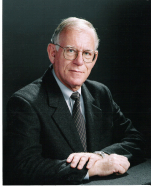# Mathematics for Physical Chemistry

5th Edition - February 1, 2023

Write a review

• Authors: Robert G. Mortimer, S. M. Blinder
• Paperback ISBN: 9780443189456

## Purchase options

Purchase options
Available for Pre-Order
Sales tax will be calculated at check-out

#### Institutional Subscription

Free Global Shipping
No minimum order

## Description

A solid understanding of mathematical principles can greatly enhance a researcher’s tool kit and understanding exactly how key mathematical concepts and methods are applied in their own field is hugely beneficial. Mathematics for Physical Chemistry specifically emphasizes the use of mathematics in the context of physical chemistry, covering foundational principles, advanced concepts, and practical applications. Now in its 5th edition, Mathematics for Physical Chemistry includes exercises in each chapter allowing readers to test their learning and put theory into practice. The early chapters are constructed around a sequence of mathematical topics, with a gradual progression into more advanced material, before further discussion of such key mathematical skills as the analysis of experimental data and, newly to this edition, complex variables. Additional new content on Mathematica and its advanced applications has also been added. Drawing on the experience of its expert authors Mathematics for Physical Chemistry is the ideal supplementary text for practicing chemists and students who want to sharpen their mathematics skills and refine their understanding of key mathematical concepts for application across physical chemistry.

## Key Features

• Includes updated coverage of key topics, including a review of general algebra and an introduction to group theory
• Features previews, objectives, and numerous examples and problems throughout the text to aid learning
• Provides chemistry-specific instruction without the distraction of abstract concepts or theoretical issues in pure mathematics
• Includes new chapters on complex variables and Mathematica for advanced applications

Students and teachers enrolled in general through physical chemistry courses, and researchers across chemistry interested in brushing up their skills

• 1. Problem Solving and Numerical Mathematics
2. Mathematical Functions
3. Problem Solving and Symbolic Mathematics: Algebra
4. Vectors and Vector Algebra
5. Problem Solving and the Solution of Algebraic Equations
6. Differential Calculus
7. Integral Calculus
8. Differential Calculus with Several Independent Variables
9. Integral Calculus with Several Independent Variables
10. Mathematical Series
11. Functional Series and Integral Transforms
12. Differential Equations
13. Operators, Matrices, and Group Theory
14. The Solution of Simultaneous Algebraic Equations with More than Two Unknowns
15. Complex Variables
16. Probability, Statistics, and Experimental Error
17. Data Reduction and the Propagation of Errors

Appendices
A. Values of Physical Constants
B. Some Mathematical Formulas and Identities
C. Infinite Series
D. A Short Table of Derivatives
E. A Short Table of Indefinite Integrals
F. A Short Table of Definite Integrals
G. Some Integrals with Exponentials in the Integrands: The Error Function
H. Answers to Selected Numerical Exercises and Problems

## Product details

• No. of pages: 400
• Language: English
• Published: February 1, 2023
• Imprint: Elsevier
• Paperback ISBN: 9780443189456

### Robert G. MortimerRobert G. Mortimer is a Professor Emeritus of Chemistry at Rhodes College in Memphis, Tennessee. He has taught physical chemistry at Indiana University and Rhodes College for over 40 years. He has carried out both experimental and theoretical research in the area of nonequilibrium processes in fluid systems.

#### Affiliations and Expertise

Rhodes College, Memphis, TN, USA

### S. M. Blinder

S.M. Blinder is a Professor Emeritus of Chemistry and Physics at the University of Michigan, Ann Arbor, and a telecommuting senior scientist with Wolfram Research in Champaign, Illinois. His research interests within the fields of theoretical chemistry and mathematical physics have included applications of quantum mechanics to atomic and molecular structure, theory and applications of Coulomb Propagators, structure and self-energy of the electron, supersymmetric quantum mechanics, and quantum computers. He is the author of four books and over 200 journal articles in theoretical chemistry and mathematical physics.

#### Affiliations and Expertise

Professor Emeritus, Chemistry and Physics, University of Michigan, Ann Arbor, MI, USA

## Ratings and Reviews

Write a review

There are currently no reviews for "Mathematics for Physical Chemistry"